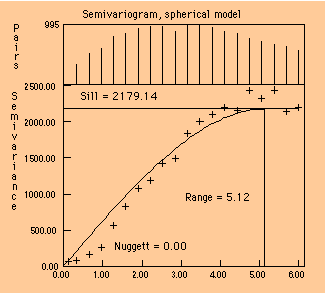## Semivariance

Regionalized variable theory does not use the autocorrelation, but instead uses a related property called the semivariance to express the degree of relationship between points on a surface. The semivariance is simply half the variance of the differences between all possible points spaced a constant distance apart.

The semivariance at a distance d = 0 should be zero, because there are no differences between points that are compared to themselves. However, as points are compared to increasingly distant points, the semivariance increases. At some distance, called the range, the semivariance will become approximately equal to the variance of the surface itself. This is the greatest distance over which the value at a point on the surface is related to the value at another point. The range defines the maximum neighborhood over which control points should be selected to estimate a grid node, to take advantage of the statistical correlation among the observations. In the circumstance where the grid node and the observations are spaced so that all distances exceed the range, kriging produces the same estimate as classical statistics, which is the mean.The semivariogram is a plot of semivariance as a function of distance between the observations, and is the source of information used in kriging to achieve optimal weighting functions for mapping. Kriging uses the semivariogram, or rather a mathematical model of the semivariogram, in calculating estimates of the surface at the grid nodes. These kriging estimates are best linear unbiased estimates of the surface at the specified locations, provided the surface is stationary and the correct form of the semivariogram has been determined.go back